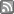# New PhD Position

The position is for three years, fully funded by Marsden grant: tuition fees and living expenses. I hope to fill this position with an able young person with an interest in one of the following two topics.

I. Consider a function-parametric empirical process $v_n(\phi)$ with functions $\phi$ from the appropriate functional space, and then apply a unitary operator to these functions, thus considering the process

$v_n(U\phi)$

Surprisingly, this opens a very fertile field of research. New mathematical structures and unusual connections have been found and more interesting work lies ahead. But leaving that to one side, from a practical" point of view one can now construct wide classes of asymptotically distribution free statistical tests for families of distributions with covariates, for classes of transition matrices of Markov chains, for parametric models for point processes, and other objects that I can not see clearly yet. The papers to read are Khmaladze(AS,2013), Khmaladze(Bernoulli, 2016), TMT Nguyen(Metrica, 2016)

The student will be asked to learn the theory of linear spaces and linear operators, the theory of empirical processes, and also such a widely used topic as martingale theory for point processes with application to survival analysis, demography, life insurance, medical insurance.

It is work which ranges from completely mathematical studies to practically useful applied statistical modeling.

II. Consider a set-valued function: for each $t\in[a,b]$ I give you a set $K(t)$ -- so, I gave you a set-valued function on $[a,b]$. Suppose this function is continuous (say, in Hausdorf metric). Is it also differentiable?, and what the derivative will be?

Set-valued analysis is old and well-developed field. There are several approaches to differentiation of set-valued functions; some of key words are "tangent cones", "(semi-, quasi-) affine mappings", "differential inclusions". However, relatively recently, we were led to define a new approach to differentiation and developed the theory for the so-called fold-up derivative". Review paper on this work was invited to AISM for publication in 2017.

To get some intuitive feeling, imagine a "good" set, $K(t_n)$ which "nicely" converges to the set $K(t)$, when $t_n$ converges to $t$. You take the difference between $K(t_n)$ and $K(t)$ -- which difference, by the way?, there are several,-- and then .. what do you do then?

Fold-up derivatives give us a very natural way to study infinitesimal changes in images. There are several approaches to analyze evolutions of images in time. Two of them are widely known -- the approach of U. Grenander and the one by D. Kendall. To compare this great work with fold-up derivatives was never attempted; but it must be extremely interesting thing to do.

Material to look at is wast. We only briefly refer to J-P Auben and H. Frankovska Set-valued Analysis", U. Grenander Statistical geometry: A tool for pattern analysis", D. Kendall et al. Shape and Shape Theory" and for fold-up derivative see E. Khmaladze, JMAA, 2007 and E. Khmaladze and W. Weil (temporary link here).

If you are interested and want to know more, let me know.

This topic: Users/Estate > NewPhDPosition
Topic revision: 15 Dec 2016, estate
Contact Us | Section Map | Disclaimer |RSS FeedBack to top ^

Valid XHTML and CSS | Built on Foswiki

Page Updated: 15 Dec 2016 by estate. © Victoria University of Wellington, New Zealand, unless otherwise stated